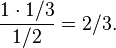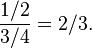You are currently browsing the tag archive for the ‘mathematics’ tag.

Wikipedia calls this a classic paradox of elementary probability theory.

Taken from Wikipedia.org:

## Card version

Suppose you have three cards:

• black card that is black on both sides,
• white card that is white on both sides, and
• mixed card that is black on one side and white on the other.

You put all of the cards in a hat, pull one out at random, and place it on a table. The side facing up is black. What are the odds that the other side is also black?

The answer is that the other side is black with probability 2/3. However, common intuition suggests a probability of 1/2 because across all the cards, there are 3 white, 3 black. However, many people forget to eliminate the possibility of the “white card” in this situation (i.e. the card they flipped CANNOT be the “white card” because a black side was turned over).

In a survey of 53 Psychology freshmen taking an introductory probability course, 35 incorrectly responded 1/2; only 3 students correctly responded 2/3.

### Preliminaries

To solve the problem, either formally or informally, we must assign probabilities to the events of drawing each of the six faces of the three cards. These probabilities could conceivably be very different; perhaps the white card is larger than the black card, or the black side of the mixed card is heavier than the white side. The statement of the question does not explicitly address these concerns. The only constraints implied by the Kolmogorov axioms are that the probabilities are all non-negative, and they sum to 1.

The custom in problems when one literally pulls objects from a hat is to assume that all the drawing probabilities are equal. This forces the probability of drawing each side to be 1/6, and so the probability of drawing a given card is 1/3. In particular, the probability of drawing the double-white card is 1/3, and the probability of drawing a different card is 2/3.

In our question, however, you have already selected a card from the hat and it shows a black face. At first glance it appears that there is a 50/50 chance (ie. probability 1/2) that the other side of the card is black, since there are two cards it might be: the black and the mixed. However, this reasoning fails to exploit all of your information; you know not only that the card on the table has a black face, but also that one of its black faces is facing you.

### Solutions:

#### Intuition

Intuition tells you that you are choosing a card at random. However, you are actually choosing a face at random. There are 6 faces, of which 3 faces are white and 3 faces are black. Two of the 3 black faces belong to the same card. The chance of choosing one of those 2 faces is 2/3. Therefore, the chance of flipping the card over and finding another black face is also 2/3. Another way of thinking about it is that the problem is not about the chance that the other side is black, it’s about the chance that you drew the all black card. If you drew a black face, then it’s twice as likely that that face belongs to the black card than the mixed card.

#### Labels

One solution method is to label the card faces, for example numbers 1 through 6. Label the faces of the black card 1 and 2; label the faces of the mixed card 3 (black) and 4 (white); and label the faces of the white card 5 and 6. The observed black face could be 1, 2, or 3, all equally likely; if it is 1 or 2, the other side is black, and if it is 3, the other side is white. The probability that the other side is black is 2/3.

#### Bayes’ theorem

Given that the shown face is black, the other face is black if and only if the card is the black card. If the black card is drawn, a black face is shown with probability 1. The total probability of seeing a black face is 1/2; the total probability of drawing the black card is 1/3. By Bayes’ theorem, the conditional probability of having drawn the black card, given that a black face is showing, is$\frac{1\cdot1/3}{1/2}=2/3.$

#### Eliminating the white card

Although the incorrect solution reasons that the white card is eliminated, one can also use that information in a correct solution. Modifying the previous method, given that the white card is not drawn, the probability of seeing a black face is 3/4, and the probability of drawing the black card is 1/2. The conditional probability of having drawn the black card, given that a black face is showing, is$\frac{1/2}{3/4}=2/3.$

#### Symmetry

The probability (without considering the individual colors) that the hidden color is the same as the displayed color is clearly 2/3, as this holds if and only if the chosen card is black or white, which chooses 2 of the 3 cards. Symmetry suggests that the probability is independent of the color chosen. (This can be formalized, but requires more advanced mathematics than yet discussed.)

#### Experiment

Using specially constructed cards, the choice can be tested a number of times. By constructing a fraction with the denominator being the number of times “B” is on top, and the numerator being the number of times both sides are “B”, the experimenter will probably find the ratio to be near 2/3.

Note the logical fact that the B/B card contributes significantly more (in fact twice) to the number of times “B” is on top. With the card B/W there is always a 50% chance W being on top, thus in 50% of the cases card B/W is drawn, card B/W virtually does not count. Conclusively, the cards B/B and B/W are not of equal chances, because in the 50% of the cases B/W is drawn, this card is simply “disqualified”.Here's Monty!

First, visit — Monty Hall Problem. Don’t read past ‘Solution.’

Attempt to solve. Write down method of solution.

Carry out ‘Simulation.’

I evidentlly prefer a more philosophical mathematical mindset.A visual of a Cantor Set

Step One: Gentle intro

“Are rational numbers countable?”

Step Two: The Hotel Infinity

“Related to Cantor’s argument that rational numbers are countable?”

Step Three: Self Similarity

“Can you write out details of constructing the Cantor Set?”